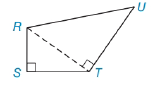Chapter 5.4, Problem 33EElementary Geometry For College St...

7th Edition
Alexander + 2 others
ISBN: 9781337614085

Solutions

Chapter
SectionElementary Geometry For College St...

7th Edition
Alexander + 2 others
ISBN: 9781337614085
Textbook Problem

In quadrilateral RSTU, R S ¯ ⊥ S T ¯ and U T ¯ ⊥ diagonal R T ¯ . If RS=6, ST= 8, and RU = 15, determine UT.To determine

To Find:

The Length of UT.

Explanation

Given In quadrilateral RSTU, RS¯ST¯ and UT¯ diagonal RT¯. If RS=6, ST= 8, and RU = 15,

From the given data,

RS = 6, ST = 8 and RU =15.

From the figure,

RS¯ST¯ and UT¯RT¯.

So RST is a right triangle, then

From the Pythagorean Theorem,

RT2=RS2+ST2RT2=(6)2+(

Still sussing out bartleby?

Check out a sample textbook solution.

See a sample solution

The Solution to Your Study Problems

Bartleby provides explanations to thousands of textbook problems written by our experts, many with advanced degrees!

Get Started

Expand each expression in Exercises 122. (2x3)(2x+3)

Finite Mathematics and Applied Calculus (MindTap Course List)

In Exercises 47-52, find and simplify f(a+h)f(a)h (h 0) for each function. 47. f(x) = x2 + 1

Applied Calculus for the Managerial, Life, and Social Sciences: A Brief Approach